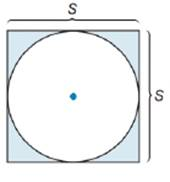Chapter 8.5, Problem 32E### Elementary Geometry for College St...

6th Edition
Daniel C. Alexander + 1 other
ISBN: 9781285195698

#### Solutions

Chapter
Section### Elementary Geometry for College St...

6th Edition
Daniel C. Alexander + 1 other
ISBN: 9781285195698
Textbook Problem
1 views

# Determine a formula for the area of the shaded region determined by the square and its inscribed circle.To determine

To find:

A formula for area of shaded region.

Explanation

Formula:

Area of circle:

If r is the radius of circle, then area of circle is given by the formula:

Acircle=πr2.

Area of square:

If the length of side of a square is a, then the area of square is given by the formula:

Asquare=a2.

Calculation:

The area of shaded region can be determined by subtracting the area of inscribed circle from the area of square.

The length of side of square is given as s.

Thus, Asquare=s2.

From the diagram, it is clear that the diameter of inscribed circle is equal to the side of square.

Diameter = s

We know that, radius of a circle is half of the length of diameter.

Thus, radius r of inscribed circle = s2

Let’s substitute the value of r in the formula to find the area of circle

### Still sussing out bartleby?

Check out a sample textbook solution.

See a sample solution

#### The Solution to Your Study Problems

Bartleby provides explanations to thousands of textbook problems written by our experts, many with advanced degrees!

Get Started

#### Solve the equations in Exercises 126. 2(x21)x2+1x4x2+1x2+1=0

Finite Mathematics and Applied Calculus (MindTap Course List)

#### In Exercises 4144, determine whether the statement is true or false. 42. 5 5

Applied Calculus for the Managerial, Life, and Social Sciences: A Brief Approach

#### True or False: If f(x) = F(x), then baf(x)dx=F(b)F(a).

Study Guide for Stewart's Single Variable Calculus: Early Transcendentals, 8th